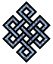#fail2ban bad ip database: ip 119.28.130.211

### | ip database | live view | stats | report | help | api key:

 ip: 119.28.130.211 hostname: 119.28.130.211 country:[CN] China first reported: 14.11.2018 08:41.12 GMT+0200 last reported: 03.12.2018 06:20.35 GMT+0200 time period: 18d 21h 39m 23s total reports: 11 reported by: 2 host(s) filter(s): ssh (9) ssh (2) tor exit node no badips.com db Lookup## port scan of '119.28.130.211':

[-hide]
```# Nmap 6.40 scan initiated Wed Nov 14 08:42:02 2018 as: /usr/bin/nmap -sU -sS -O 119.28.130.211
Nmap scan report for 119.28.130.211
Host is up (0.20s latency).
Not shown: 1000 open|filtered ports, 989 filtered ports
PORT     STATE  SERVICE
22/tcp   open   ssh
80/tcp   open   http
443/tcp  open   https
1234/tcp open   hotline
2222/tcp closed EtherNet/IP-1
3306/tcp open   mysql
3389/tcp closed ms-wbt-server
7000/tcp closed afs3-fileserver
8080/tcp open   http-proxy
9001/tcp closed tor-orport
9009/tcp closed pichat
No exact OS matches for host (If you know what OS is running on it, see http://nmap.org/submit/ ).
TCP/IP fingerprint:
OS:SCAN(V=6.40%E=4%D=11/14%OT=22%CT=2222%CU=%PV=N%G=Y%TM=5BEBD1FC%P=x86_64-
OS:pc-linux-gnu)SEQ(SP=108%GCD=1%ISR=10C%TI=Z%CI=I%TS=8)SEQ(SP=107%GCD=1%IS
OS:R=10A%TI=Z%TS=8)OPS(O1=M590ST11NW7%O2=M590ST11NW7%O3=M590NNT11NW7%O4=M59
OS:0ST11NW7%O5=M590ST11NW7%O6=M590ST11)WIN(W1=7120%W2=7120%W3=7120%W4=7120%
OS:W5=7120%W6=7120)ECN(R=Y%DF=Y%TG=40%W=7210%O=M590NNSNW7%CC=Y%Q=)T1(R=Y%DF
OS:=Y%TG=40%S=O%A=S+%F=AS%RD=0%Q=)T2(R=N)T3(R=N)T4(R=Y%DF=Y%TG=40%W=0%S=A%A
OS:=Z%F=R%O=%RD=0%Q=)T5(R=Y%DF=Y%TG=40%W=0%S=Z%A=S+%F=AR%O=%RD=0%Q=)T6(R=Y%
OS:DF=Y%TG=40%W=0%S=A%A=Z%F=R%O=%RD=0%Q=)T7(R=Y%DF=Y%TG=40%W=0%S=Z%A=S+%F=A
OS:R%O=%RD=0%Q=)U1(R=N)IE(R=Y%DFI=N%TG=40%CD=S)

OS detection performed. Please report any incorrect results at http://nmap.org/submit/ .
# Nmap done at Wed Nov 14 08:42:52 2018 -- 1 IP address (1 host up) scanned in 50.12 seconds
```
```Σ = 50 | Δt = 0.0034990310668945s
```